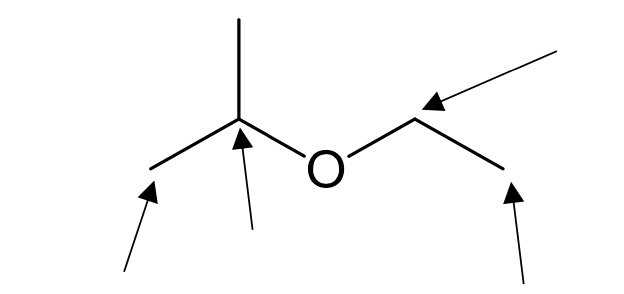top of page
Search

# Determine the multiplicity of indicated signals in the expected 1H NMR spectrum of the following com

Determine the multiplicity of indicated signals in the expected 1H NMR spectrum of the following compound.Signal Splitting and the (n+1) Rule

The n+1 rule predicts the splitting pattern for hydrogens on the HNMR graph, where n is the number of neighboring hydrogens.Determine the multiplicity of indicated signals in the expected 1H NMR spectrum of the following compound.

The 3 hydrogens on the left have 1 neighboring hydrogen and are therefore a doublet (1+1=2). 1 Hydrogen in the middle has 6 neighboring hydrogens and is therefore a septet (6+1=7). 2 hydrogens on the right have 3 neighboring hydrogens and are a quartet (3+1). 3 hydrogens on the right have 2 neighboring hydrogens and are a triplet (2+1=3).